# Multiplying Fractions by Simplifying First

Multiplying Fractions by Simplifying First• Before multiplying these fractions we can simplify the calculation by cancelling.
• We look for a number that divides exactly into both a number on the top and a number on the bottom.
• We can see that both 2 and 16 are even numbers and can be divided by 2.
• We can halve 2 to get 1 and halve 16 to get 8.
• Because we have halved a number on the top and halved a number on the bottom, the answer will be the same.
•   2 / 7   ×   1 / 16   is the same as   1 / 7   ×   1 / 8  .
• Now that we have simplified the fractions we do the multiplication.
• To multiply fractions we multiply the together and then multiply the together separately.
• 1 × 1 = 1 and 7 × 8 = 56.
•   1 / 7   ×   1 / 8   =   1 / 56  .

Look for a number on the top and a number on the bottom that can both be divided by the same number.

Divide them both by this number before multiplying the fractions.• We look for a number on the top and number on the bottom that can both be divided by the same number.
• 5 and 10 are both in the 5 times table so can be divided by 5.
• 5 ÷ 5 = 1 and 10 ÷ 5 = 2.
• We can also see that 9 and 12 are both in the 3 times table and can be divided by 3.
• 9 ÷ 3 = 3 and 12 ÷ 3 = 4.
•   5 / 12   ×   9 / 10   is the same as   1 / 4   ×   3 / 2  .
• Multiplying the numerators, 1 × 3 = 3.
• Multiplying the denominators, 4 × 2 = 8.
•   5 / 12   ×   9 / 10   =   3 / 8  .Supporting Lessons# How to Multiply Fractions by Simplifying First

## Multiplying Fractions by Simplifying First

To multiply fractions by simplifying first, use the following steps.

• Find a number on top of the fractions and a number on the bottom of the fractions that can both be divided by the same number.
• Divide both numbers in the fractions by this number.
• Write the answers to this division in the place of the original numbers in the fraction.
• Multiply the fractions like usual by multiplying the numerators and denominators separately.
• Multiplying the numerators on top of the fractions equals the numerator on the top of the answer.
• Multiplying the denominators on the bottom of the fractions equals the denominator on the bottom of the answer.

Here is an example of simplifying fractions before multiplying.

Here we have   4 / 15   ×   5 / 9  .

The first step is to look for a number on the top of either of the fractions and a number on the bottom of either of the fractions that can both be divided by the same number.

We can see that both 5 on the top of the right fraction and 15 on the bottom of the left fraction are both in the 5 times table.

We say that 5 is a factor of both 5 and 15. A factor is a number that divides exactly into another number.

So we divide both 5 and 15 by 5.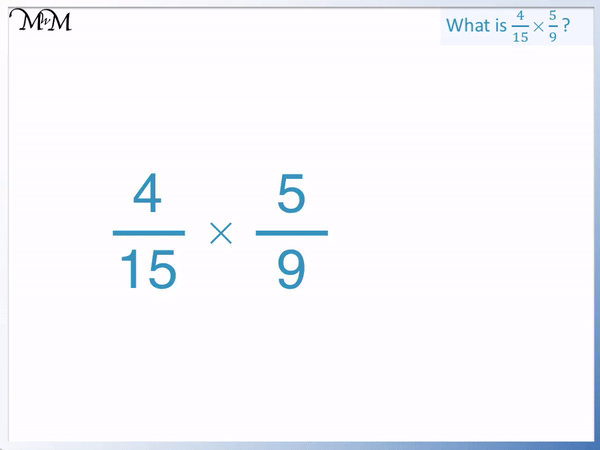5 ÷ 5 = 1 and 15 ÷ 5 = 3.

We cross out the 5 and 15 and replace them with a 1 and a 3.

Now that we have simplified the fractions we can multiply them.

To multiply fractions, simply multiply the numerators and denominators separately.

The numerators are the numbers on the top.

4 × 1 = 4

The denominators are the numbers on the bottom.

3 × 9 = 27

4 / 15   ×   5 / 9   =   4 / 27  .

We know that the answer is fully simplified because no numbers divide into both 4 and 27.

Dividing the top and bottom of a fraction by the same number can be called cancelling the fraction.

When we cross off a number on the top of one fraction and the number on the bottom of another fraction diagonally, we can call it cross cancelling.

## Why does Cross Cancelling Work?

We multiply by numbers on the top of fractions and divide by numbers on the bottom of fractions. This is because the line in a fraction means to divide by the number below it.

Cross cancelling divides the number we are multiplying by and the number we are dividing by by the same amount. This means that the answer does not change in size. The values have been multiplied by less but also divided by less so the answer remains the same as it would have before cross cancelling.

For example, here we have the multiplication of   2 / 7   ×   1 / 16  .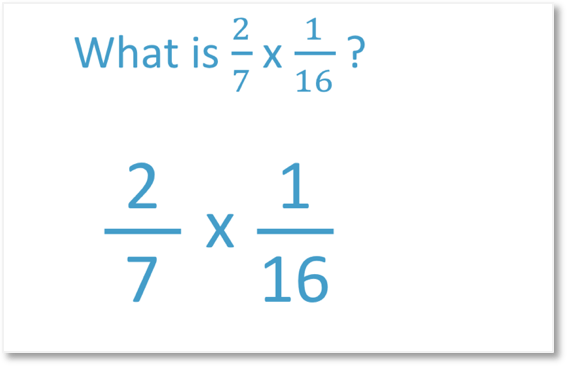We can multiply the fractions without simplifying first.

We multiply the numerators on top first.

2 × 1 = 2

And multiply the denominators on the bottom.

7 × 16 = 112

Therefore   2 / 7   ×   1 / 16   =   2 / 112  .

We can simplify the fraction afterwards by dividing both the numerator on top and the denominator on the bottom by 2.

2 / 112   =   1 / 56  , which is our final answer.

We simplified our answer by dividing the top and bottom by 2, just at the end of our process.

Instead we can simplify the fraction first by cross cancelling.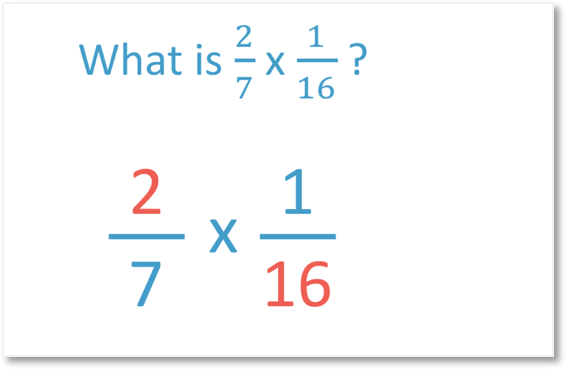The number 2 and the number 16 both can be divided by 2. This time, we will divide the top and the bottom by 2 before we multiply. 2 is a common factor of 2 and 16.

2 ÷ 2 = 1 and 16 ÷ 2 = 8.Multiplying the fractions   1 / 7   ×   1 / 8   =   1 / 56  .

This gives us the same answer as before.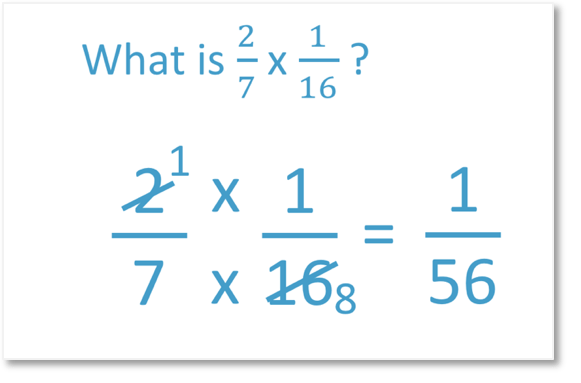We can also think of fractions as a multiplication and division sentence. We multiply by the numerators on top and divide by the denominators on the bottom.

2 / 7   ×   1 / 16   is the same as 2 × 1 ÷ 7 ÷ 16.

1 / 7   ×   1 / 8   is the same as 1 × 1 ÷ 7 ÷ 8.

We can compare the underlined digits to see that we have halved the number we are multiplying by from 2 to 1 but also halved the number we are dividing by from 16 to 8. The answer is the same.## Why do we Simplify Fractions before Multiplying Them?

Multiplying some fractions can result in large numbers being multiplied together. It is best to simplify fractions first by cross cancelling common factors to make the numbers smaller. Smaller numbers are easier to multiply.

You are also less likely to make a mistake by simplifying the fractions first. Even the final step of simplifying is easier because the numbers are smaller. Multiplying the fractions without simplifying first may result in very large numbers and it may not be obvious what to divide them by to simplify the fraction.

For example, here is   5 / 12   ×   9 / 10  .

5 × 9 = 45 and 12 × 10 = 120

5 / 12   ×   9 / 10   =   45 / 120  .

The numbers in the fraction were not particularly easy to multiply but the final step of simplifying the fraction is not easy as it is hard to see the highest common factor that divides into both 45 and 120.

In fact, both 45 and 120 can be divided by 15.

45 ÷ 15 = 3 and 120 ÷ 15 = 8.

However it is much easier to simplify the fractions before multiplying.We can see that both 5 and 10 can be divided by 5 and 9 and 12 can be divided by 3.

5 / 12   ×   9 / 10   =   1 / 4   ×   3 / 2  .

This results in much easier numbers to multiply and there is then no need to simplify a large fraction as the end.

1 / 4   ×   3 / 2   =   3 / 8  .

Here is another example of why we simplify fractions before multiplying them.

We have   9 / 14   ×   4 / 15  .

Again multiplying the fraction immediately can result in larger numbers.

9 × 4 = 36 and 14 × 15 = 210

9 / 14   ×   4 / 15   =   36 / 210   which is not so easy to simplify.

We can divide by the common factor of 6 in 36 and 210.

36 ÷ 6 = 6 and 210 ÷ 6 = 35.

However it is much easier to simplify the fraction first to keep the numbers within the usual times tables.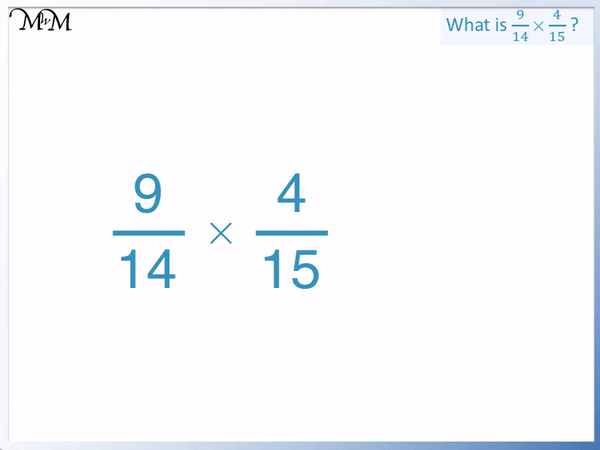We can divide both 9 and 15 by 3 to get 3 and 5 respectively.

We can also divide 4 and 14 by 2 to get 2 and 7 respectively.

3 / 7   ×   2 / 5   =   6 / 35  .

Because we already fully simplified the fractions first before multiplying them, there is no need to simplify our answer again. It is already in its simplest form.Now try our lesson on Converting Fractions to Percentages where we learn how to write a fraction as a percentage.error: Content is protected !!